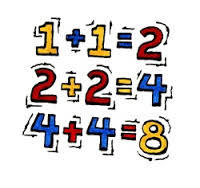3 years ago
bertile
Save
Edit
Host a game
Live GameLive
Homework
Solo Practice
Practice10 QuestionsShow answers
• Question 1
30 seconds
Q. 2+2=
1
4
3
5
• Question 2
30 seconds
Q. 1+1=
2
0
4
1
• Question 3
30 seconds
Q. 3+3=
4
5
6
3
• Question 4
30 seconds
Q. 4+4=
5
2
6
8
• Question 5
30 seconds
Q. 5+5=
6
10
8
9
• Question 6
30 seconds
Q. 6+6=
12
10
21
8
• Question 7
30 seconds
Q. 7+7=
14
12
10
20
• Question 8
30 seconds
Q. 8+8=
11
10
16
14
• Question 9
30 seconds
Q. 9+9=
15
18
12
16
• Question 10
30 seconds
Q. 10+10=